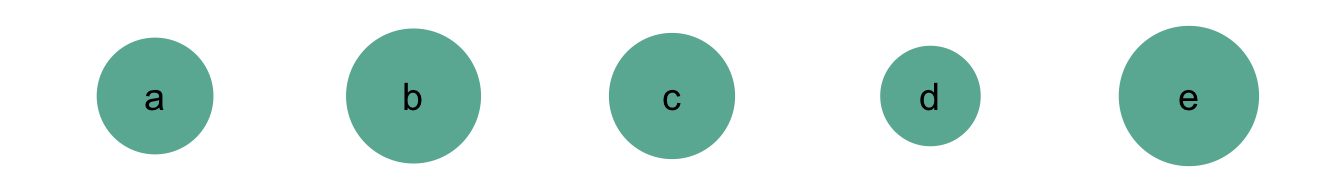The human eye does not perform well when it has to translate areas to numeric values. Let’s consider the following five bubbles. Try to rank them by decreasing area. You will probably agree that this is possible, but takes some time.

``````# Libraries
library(tidyverse)
library(hrbrthemes)

# create 3 data frame:
data <- data.frame( name=letters[1:5], value=c(17,24,20,15,27) )

# Plot
ggplot(data, aes(x=name, y=1, size=value)) +
geom_point(color="#69b3a2") +
geom_text(aes(label=name), size=5) +
scale_size_continuous(range=c(17,24)) +
theme_void() +
theme(
legend.position="none"
) +
ylim(0.9,1.1)``````Now, let’s represent the exact same values using bars instead:

``````# Plot
ggplot(data, aes(x=name, y=value)) +
geom_bar(stat="identity", fill="#69b3a2") +
theme_ipsum()``````